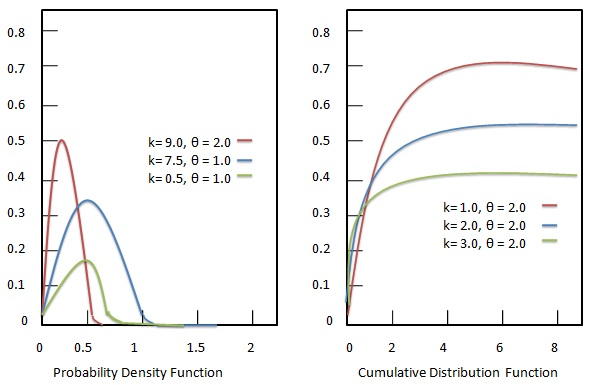# Statistics – Gamma Distribution# Statistics – Gamma Distribution

The gamma distribution represents continuous probability distributions of two-parameter family. Gamma distributions are devised with generally three kind of parameter combinations.

• A shape parameter kk and a scale parameter θθ.
• A shape parameter α=kα=k and an inverse scale parameter β=1θβ=1θ, called as rate parameter.
• A shape parameter kk and a mean parameter μ=kβμ=kβ.Each parameter is a positive real numbers. The gamma distribution is the maximum entropy probability distribution driven by following criteria.

## FormulaWhere −

• XX = Random variable.
• ψψ = digamma function.

## Characterization using shape αα and rate ββ

### Probability density function

Probability density function of Gamma distribution is given as:

## FormulaWhere −

• αα = location parameter.
• ββ = scale parameter.
• xx = random variable.

### Cumulative distribution function

Cumulative distribution function of Gamma distribution is given as:

## FormulaWhere −

• αα = location parameter.
• ββ = scale parameter.
• xx = random variable.
• γ(α,βx)γ(α,βx) = lower incomplete gamma function.

## Characterization using shape kk and scale θθ

### Probability density function

Probability density function of Gamma distribution is given as:

## Formula